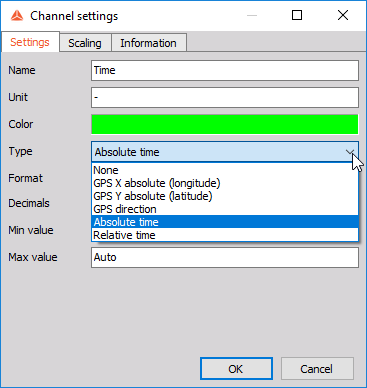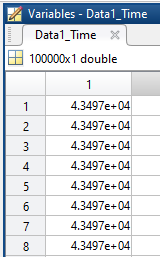When 'absolute time' channels are used in Dewesoft, they can be presented in different formats. The default format is presented in 'excel' time format.

For example we created a formula which uses 'localabstime', which presents local absolute time:Values of the formula are shown in the Digital meter. Representation will differ depending on which setting is chosen. The setting can be seen in the following picture:If the 'default' option is selected the value will be presented in 'excel' time format and if we select Absolute time then the value will be presented in 'Absolute' time format:When we export the channel to Matlab, the channel's values will be exported in the default, 'excel' time format:If we want to convert the values to 'Absolute' time format we need to call the following function:

t = datetime ( Data1_Time, 'ConvertFrom', 'excel')

The values are then presented in 'Absolute' time format, which is more easier to read: